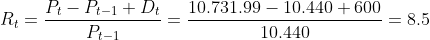Scan QR code or get instant email to install app

Question:

# An investor purchased a $10,000, 5-year corporate note one year ago for$10,440. The note pays an annual coupon of \$600. Over the past year, the note’s annual yield-to-maturity has dropped by 1%. What total return did the investor earn over the year?

A 8.5%.
explanation

1212First, find the yield on the note at time of purchase. The appropriate calculator steps are:
PV =-10,440; FV = 10,000; PMT = 600; N = 5; CPT -> I/Y = 4.9842%. Next, value the note at a yield of 3.9842% with four years to maturity.
FV = 10,000; PMT = 600; N = 4; I/Y = 3.9842; CPT -> PV = 10,731,99.
Finally, calculate the holding period return. The formula is: